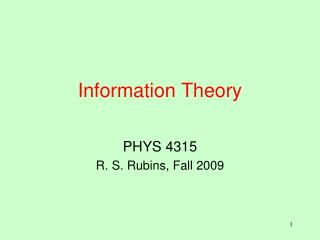DownloadDownload PresentationInformation Theory

# Information Theory

Download Presentation## Information Theory

- - - - - - - - - - - - - - - - - - - - - - - - - - - E N D - - - - - - - - - - - - - - - - - - - - - - - - - - -
##### Presentation Transcript

1. Information Theory PHYS 4315 R. S. Rubins, Fall 2009

2. Lack of Information • Entropy S is a measure of the randomness (or disorder) of a system. • A quantum system in its single lowest state is in a state of perfect order: S=0 (3rd law). • A system at higher temperatures may be in one of many quantum states, so that there is a lack of information about the exact state of the system. • The greater the lack of information, the greater is the disorder. • Thus, a disordered system is one about which we lack complete information. • Information theory (Shannon, 1948) provides a mathematical measure of the lack of information, which may be linked to the entropy.

3. Missing Information H • For an experiment with n possible outcomes p1, p2,… pn, Shannon introduced a function H(p1, p2,… pn), which quantitatively measures the missing information associated with the set of probabilities. • Three conditions are needed to specify H to within a constant factor. • 1. H is a continuous function of pi. • 2. If all the pi are equal, then pi = 1/n, and H is a monotonically increasing function of n, since the number of possibilities increases with n. • 3. If the possible outcomes of an experiment depend on the outcomes of n subsidiary experiments, then H is the sum of the uncertainties of the subsidiary experiments. • With these assumptions, H was found to be proportional to the entropy S = – k r pr ln pr.

4. Example of Sum of Uncertainties Single experiment, using H = – K(pr lnpr). H(1/2,1/3,1/6) = – K[(1/2) ln(1/2) + (1/3) ln(1/3) + (1/6) ln(1/6)] = K[(1/2)ln2 + (1/3)ln3 + (1/6)ln6] = K[(2/3)ln2 + (1/2)ln3] = 1.01 K. Two successive experiments H(1/2,1/2) = – K[(1/2) ln(1/2) + (1/2) ln(1/2)] = K ln2. (1/2)H(2/3,1/3) = K[(– (1/3)ln2 + (1/3)ln3 + (1/6)ln3] Thus, H(1/2,1/2) + (1/2)H(2/3,1/3) = K{[1 – (1/3)]ln2 + [(1/3) + (1/6)]ln3} = K[(2/3)ln2 + (1/2)ln3] = 1.01 K.

5. Shannon’s Calculation 1 The simplest choice of continuous function (Condition 1)is For simplicity, consider the case of equal probabilities; i.e. pi = 1/n. Since H is a monotonically increasing function of n (Condition 2), For two successive experiments (Condition 3)

6. Shannon’s Calculation 2 Since H(1/n,…1/n) = n f(1/r), . Letting R = 1/r and S =1/s,

7. Shannon’s Calculation 3 Since g(R) + g(S) = g(R,S) and , so that Thus, so that Since R = 1/r, .

8. Shannon’s Calculation 4 For r =1, the result is certain, so that H(r) = f(1/r) = 0. Thus, C =0, so that Now d/dn(– A ln n) must be positive (Condition 2), so that A must be negative. Letting K = – A, and p = pi =1/n, Thus, the missing information function H is given by . With K replaced by Boltzmann’s constant k, H equals S (entropy).